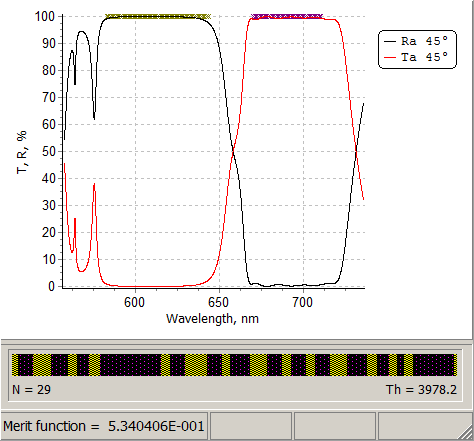### Stress and thickness targets

Optimization of total thickness of one coating material

Minimization of the total coating thickness

Reducing of stress using alignment of thicknesses of materials on front and back sides

Alignment of stresses caused by front- and backside coatings

Auxiliary information for stress-targets specification

New type of target implemented in Version 13.77 allows you to optimize linear combinations of thicknesses of coating materials.

Using this option you can optimize, for example,  total thicknesses of materials of your design, minimize stresses, optimize total coating thickness. This possibility is available for all OptiLayer structures: one-side coatings, for double-side coatings, and for stacks.

The new option is extremely flexible and powerful and opens a new horizon in multi-criteria design problems.Fig. 1. Possible expressions for optimization.Fig. 2. Thickness and stress target.

Thickness/Stress target(s) can be specified for all coatings: front side, front/back sides, surfaces in a stack.  The maximal number of coatings is equal to maximal number of active surfaces.

You can specify multiple targets. The number of targets is specified in Thickness/Stress Target number.

Below we show several examples helping you to get experience with this powerful and flexible option.

### Example 1. Optimization of total thickness of one of the coating materials.

Consider a design problem of edge filters, which exhibit high reflectance in the range 583-683 nm and high transmittance in the range 670-710 nm, AOI is 45°. Assume that two typical thin-film materials, TiO2 and SiO2, are used.

Multiple design solutions can be obtained using needle optimization, gradual evolution, random optimization.

Typical design solutions contain 25-30 layers and have total thickness of 3.6-4 μm (Fig. 3). Total thickness of TiO2 layers varies from 1.2 to 2.1 μm. Merit function values are 0.4-0.8.

It may be required to reduce the total thickness of TiO2 layers since TiO2 can be slightly absorbing. To achieve this, stress/thickness targets can be used.

A realistic requirements would be to limit the TiO2 thickness by 1 μm, i.e.:

$\Sigma_{TiO_2}\le 1\mu m$Fig. 3. Spectral characteristics of conventional edge filter design solutions. The number of layers N is 25-30, total thickness is between 3.6-4 μm, merit function values 0.4-0.8.

Stress/thickness target can be specified as it is shown in Fig. 2. Coefficients $$\alpha_1$$ and $$\beta_1$$ take the values 0 and 1, respectively. The target value is 1000, that corresponds to 1000 nm. If thickness units were μm instead of nm, the target value would be equal to 1.

Thick/Stress target can be loaded along with the conventional edge filter target. Optimization methods will operate in the usual way.

One of the possible solutions (Fig. 4 and Fig. 5) contains 23 layers and has total thickness of 3.5 μm.Fig. 4. Spectral curves of a design solution obtained as result of merit function optimization with respect to two targets (conventional and Thick/Stress.Fig. 5. Refractive index profile of a 23-layer design solution obtained with optimization of convenient and Thick/Stress targets simultaneously.

Refractive index profile (Fig. 5) shows that relatively thick SiO2 layers appeared. This is not surprising since the design optical thickness is very conservative parameter. Therefore, the algorithm tries to keep up the total optical thickness of the design.

Total thickness of TiO2 layers in the solution is now 1.026 μm.

Total thickness of SiO2 layers is this solution is about 2.5 μm.

Comparison of separate values of merit function composition responsible for the conventional and stress/thickness targets (numerical values in Fig. 6 and diagram in Fig. 7) shows that the requirement on TiO2  thickness is completely satisfied: stress/thickness component is close to zero.Fig. 6. Total merit function value and two components (conventional and Thick/Stress ones).Fig. 7. Two components of the merit function (conventional and Thick/Stress ones) on the diagram.

Attempts to reduce thicknesses of thick SiO2 layers will lead to reduction of the total thickness and as a consequence to worsening of the approximation of target spectral characteristics. A thinner 23-layer design solution exhibits not perfect spectral performance (Fig. 8). Total thickness of TiO2 layers is 1.031 μm, total thickness of SiO2 layers is about 2 μm.Fig. 8. Spectral curves of a thinner 23-layer edge filter. Merit function is two times larger than the merit function of the edge filter above.Fig. 9. Refractive index profile of a thinner 23-layer edge filter obtained using optimization of convenient and Thick/Stress targets simultaneously.

Auxiliary information for stress-targets specification